﻿ 前端面试的底气之实现一个深拷贝 - 自由资讯

# 前端面试的底气之实现一个深拷贝

• 前言
• 青铜段位
• 白银段位
• 黄金段位
• 铂金段位
• 砖石段位
• 星耀段位
• 王者段位
• 总结

## 青铜段位

`JSON.parse(JSON.stringify(data))`

• 如果对象中存在循环引用的情况也无法正确实现深拷贝。
```const a = {
b: 1,
}
a.c = a;
JSON.parse(JSON.stringify(a));```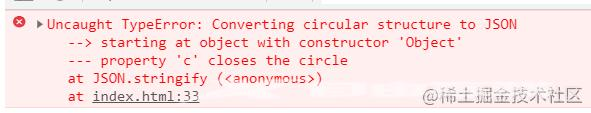• 如果 data 里面有时间对象，则`JSON.stringify`后再`JSON.parse`的结果，时间将只是字符串的形式。而不是时间对象。
```const a = {
b: new Date(1536627600000),
}
console.log(JSON.parse(JSON.stringify(a)))```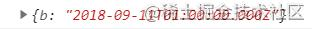• 如果 data 里有RegExp、Error对象，则序列化的结果将只得到空对象；
```const a = {
b: new RegExp(/\d/),
c: new Error('错误')
}
console.log(JSON.parse(JSON.stringify(a)))```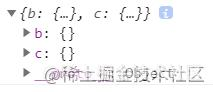• 如果 data 里有函数，undefined，则序列化的结果会把函数置为undefined或丢失；
```const a = {
b: function (){
console.log(1)
},
c:1,
d:undefined
}
console.log(JSON.parse(JSON.stringify(a)))```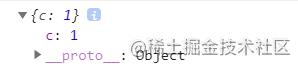• 如果 data 里有NaN、Infinity和-Infinity，则序列化的结果会变成null
```const a = {
b: NaN,
c: 1.7976931348623157E+10308,
d: -1.7976931348623157E+10308,
}
console.log(JSON.parse(JSON.stringify(a)))```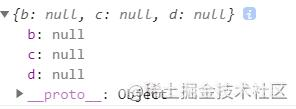## 白银段位

```function deepClone(target){
if(target !== null && typeof target === 'object'){
let result = {}
for (let k in target){
if (target.hasOwnProperty(k)) {
result[k] = deepClone(target[k])
}
}
return result;
}else{
return target;
}
}```

• 对原始类型和引用类型数据的判断能力。
• 对递归思维的应用的能力。
• 深入理解for...in的用法。

## 黄金段位

```function deepClone(target){
if(target !== null && typeof target === 'object'){
let result = Object.prototype.toString.call(target) === "[object Array]" ? [] : {};
for (let k in target){
if (target.hasOwnProperty(k)) {
result[k] = deepClone(target[k])
}
}
return result;
}else{
return target;
}
}```

• 正确理解引用类型概念的能力。
• 精确判断数据类型的能力。

## 铂金段位

```let data = {
a: 1
};
data.f=data```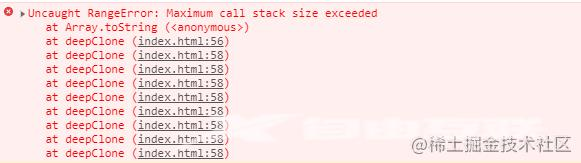```function deepClone(target) {
function clone(target, map) {
if (target !== null && typeof target === 'object') {
let result = Object.prototype.toString.call(target) === "[object Array]" ? [] : {};
if (map[target]) {
return map[target];
}
map[target] = result;
for (let k in target) {
if (target.hasOwnProperty(k)) {
result[k] = clone(target[k],map)
}
}
return result;
} else {
return target;
}
}
let map = new Map();
const result = clone(target, map);
map.clear();
map = null;
return result
}```

• 对循环引用的理解，如何解决循环引用引起的问题的能力。
• 熟悉 ES6 中 Map 数据结构的概念及应用
• 对内存泄露的认识和避免泄露的能力。

## 砖石段位

• WeakMap 只接受对象作为键名（ null 除外），不接受其他类型的值作为键名。但是 target 正好符合要求故不影响。

• WeakMap 的键名所指向的对象不计入垃圾回收机制。这里用下面的例子来解释。

```let map = new WeakMap();
let obj = {a : 1}
map.set(obj , 1);```

```function deepClone(target) {
/**
* 遍历数据处理函数
* @array 要处理的数据
* @callback 回调函数，接收两个参数 value 每一项的值 index 每一项的下标或者key。
*/
function handleWhile(array, callback) {
const length = array.length;
let index = -1;
while (++index < length) {
callback(array[index], index)
}
}
function clone(target, map) {
if (target !== null && typeof target === 'object') {
let result = Object.prototype.toString.call(target) === "[object Array]" ? [] : {};
// 解决循环引用
if (map.has(target)) {
return map.get(target);
}
map.set(target, result);

const keys = Object.prototype.toString.call(target) === "[object Array]" ? undefined : Object.keys(
target);

function callback(value, key) {
if (keys) {
// 如果keys存在则说明value是一个对象的key，不存在则说明key就是数组的下标。
key = value;
}
result[key] = clone(target[key], map)
}
handleWhile(keys || target, callback)
return result;
} else {
return target;
}
}
let map = new WeakMap();
const result = clone(target,map);
map = null;
return result
}```

```let arr = [];
for (let i = 0; i < 1000000; i++) {
arr.push(i)
}
let data = {
a: arr
};
console.time();
const result = deepClone(data);
console.timeEnd();
console.time();
const result1 = deepClone1(data);
console.timeEnd();```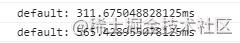• 熟悉 ES6 中 WeakMap 数据结构的概念及应用
• 具有优化代码运行性能的能力。
• 了解遍历的效率的能力。
• 了解 `++i``i++` 的区别。
• 代码抽象的能力。

## 星耀段位

• 判断数据不是引用类型时就直接返回 target，但是原始类型中还有 Symbol 这一特殊类型的数据，因为其每个 Symbol 都是独一无二，需要额外拷贝处理，不能直接返回。

• 判断数据是不是引用类型时不严谨，漏了 `typeof target === function'` 的判断。

• 只考虑了 Array、Object 两种引用类型数据的处理，引用类型的数据还有Function 函数、Date 日期、RegExp 正则、Map 数据结构、Set 数据机构，其中 Map 、Set 属于 ES6 的。

```function deepClone(target) {
// 获取数据类型
function getType(target) {
return Object.prototype.toString.call(target)
}
//判断数据是不是引用类型
function isObject(target) {
return target !== null && (typeof target === 'object' || typeof target === 'function');
}
//处理不需要遍历的应引用类型数据
function handleOherData(target) {
const type = getType(target);
switch (type) {
case "[object Date]":
return new Date(target)
case "[object RegExp]":
return cloneReg(target)
case "[object Function]":
return cloneFunction(target)

}
}
//拷贝Symbol类型数据
function cloneSymbol(targe) {
const a = String(targe); //把Symbol字符串化
const b = a.substring(7, a.length - 1); //取出Symbol()的参数
return Symbol(b); //用原先的Symbol()的参数创建一个新的Symbol
}
//拷贝正则类型数据
function cloneReg(target) {
const reFlags = /\w*\$/;
const result = new target.constructor(target.source, reFlags.exec(target));
result.lastIndex = target.lastIndex;
return result;
}
//拷贝函数
function cloneFunction(targe) {
//匹配函数体的正则
const bodyReg = /(?<={)(.|\n)+(?=})/m;
//匹配函数参数的正则
const paramReg = /(?<=\().+(?=\)\s+{)/;
const targeString = targe.toString();
//利用prototype来区分下箭头函数和普通函数，箭头函数是没有prototype的
if (targe.prototype) { //普通函数
const param = paramReg.exec(targeString);
const body = bodyReg.exec(targeString);
if (body) {
if (param) {
const paramArr = param.split(',');
//使用 new Function 重新构造一个新的函数
return new Function(...paramArr, body);
} else {
return new Function(body);
}
} else {
return null;
}
} else { //箭头函数
//eval和函数字符串来重新生成一个箭头函数
return eval(targeString);
}
}
/**
* 遍历数据处理函数
* @array 要处理的数据
* @callback 回调函数，接收两个参数 value 每一项的值 index 每一项的下标或者key。
*/
function handleWhile(array, callback) {
let index = -1;
const length = array.length;
while (++index < length) {
callback(array[index], index);
}
}
function clone(target, map) {
if (isObject(target)) {
let result = null;
if (getType(target) === "[object Array]") {
result = []
} else if (getType(target) === "[object Object]") {
result = {}
} else if (getType(target) === "[object Map]") {
result = new Map();
} else if (getType(target) === "[object Set]") {
result = new Set();
}

// 解决循环引用
if (map.has(target)) {
return map.get(target);
}
map.set(target, result);

if (getType(target) === "[object Map]") {
target.forEach((value, key) => {
result.set(key, clone(value, map));
});
return result;
} else if (getType(target) === "[object Set]") {
target.forEach(value => {
});
return result;
} else if (getType(target) === "[object Object]" || getType(target) === "[object Array]") {
const keys = getType(target) === "[object Array]" ? undefined : Object.keys(target);

function callback(value, key) {
if (keys) {
// 如果keys存在则说明value是一个对象的key，不存在则说明key就是数组的下标。
key = value
}
result[key] = clone(target[key], map)
}
handleWhile(keys || target, callback)
} else {
result = handleOherData(target)
}
return result;
} else {
if (getType(target) === "[object Symbol]") {
return cloneSymbol(target)
} else {
return target;
}
}
}
let map = new WeakMap;
const result = clone(target, map);
map = null;
return result
}```

• 代码逻辑的严谨性。
• 深入了解数据类型的能力。
• JS Api 的熟练使用的能力。
• 了解箭头函数和普通函数的区别。
• 熟练使用正则表达式的能力。
• 模块化开发的能力

## 总结

• 白银段位
• 对原始类型和引用类型数据的判断能力。
• 对递归思维的应用的能力。
• 黄金段位
• 正确理解引用类型概念的能力。
• 精确判断数据类型的能力。
• 铂金段位
• 对循环引用的理解，如何解决循环引用引起的问题的能力。
• 熟悉 ES6 中 Map 数据结构的概念及应用。
• 对内存泄露的认识和避免泄露的能力。
• 砖石段位
• 熟悉 ES6 中 WeakMap 数据结构的概念及应用。
• 具有优化代码运行性能的能力。
• 了解遍历的效率的能力。
• 了解 `++i``i++` 的区别。
• 代码抽象的能力。
• 星耀段位
• 代码逻辑的严谨性。
• 深入了解数据类型的能力。
• JS Api 的熟练使用的能力。
• 了解箭头函数和普通函数的区别。
• 熟练使用正则表达式的能力。
• 模块化开发的能力

﻿